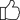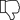Fullerton India Credit Co. Ltd. is Now SMFG India Credit Co. Ltd.

# What is a Simple Interest Rate? Meaning, Formula & How to Calculate?

Published on Sept 19, 2022Updated on Dec 5, 2023Simple interest is one of the easiest ways to calculate the annual interest expenses and income on loans and deposits. Therefore, it becomes important to know the concept of simple interest and how to calculate simple interest.

## What is Simple Interest?

Simple interest is the way to directly calculate the percentage charged on the principal amount for a specific period. One of the unique features of a simple interest calculation is the principal amount remains constant irrespective of the time period.

## Simple Interest Rate Formula

Simple Interest = Principal (P) * Rate of Interest (R)* Time (T)

To calculate the simple interest, the interest is applied to the initial principal amount and then multiplied by the time period. The time period is always denoted in years and if the time period is denoted in months, it needs to be converted to year format. For example, if the time period is 9 months, it needs to be put up as 9/12 to make it year-wise.

Let’s check how interest is calculated using a simple interest formula with the following example.

Principal amount: Rs 1 lakh
Rate of interest: 12%
Time period: 18 months
Simple Interest= 1,00,000 * (12/100) * (18/24)= Rs 9,000

As per simple interest calculation, the interest amount is Rs 9,000.

With the advent of online free calculators, you can directly calculate the simple interest amount instantly without the need to do it manually.

## Limitations of Simple Interest

Despite being one of the easiest ways to calculate interest on the principal amount, there are a few limitations, including:

• Cannot calculate the benefits of compounding: It fails to calculate progressive returns on the deposits or the loan amount. For this reason, compound interest is used to calculate the benefits of compounding accurately.
• Limited application: In modern banking and finance, simple interest has lost relevance and is only used to calculate interest on short-term (less than 12 months) deposits and loans.

## How to Calculate Interest Expenses on an Instant Personal Loan?

On a personal loan, the interest is calculated on the outstanding principal amount. Therefore, after every EMI, the interest expenses change.

For instance, if you have taken an instant personal loan of Rs 5 lakh at a 14% rate of interest for three years, your EMI amount will be Rs 17,089 per month.

In the first month, the share of interest expenses on the EMI amount will be Rs 5,833 and the principal repaid will be Rs 11,256. As the outstanding principal amount has decreased after payment of the first EMI, the interest expense also reduces. In the second month, the share of interest expenses on the EMI amount will be Rs 5,702 and the principal amount will be Rs 11,387.

Using the simple interest formula, it is difficult to accurately calculate the interest expense due to the complexities and changing principal amount and time period. Therefore, using a personal loan calculator will help you calculate the EMI amount and share of interest expense in each EMI accurately.

## What is a Personal Loan Calculator?

The personal loan calculator uses a modified version of the simple interest formula. It uses the formula:

EMI= P*R*(1+r)n/((1+n)n-1)

P= principal amount, R= rate of interest, and n= tenure in months.

The personal loan EMI calculator gives you a near-accurate estimate of your monthly EMI by taking into account the principal amount, tenure, and personal loan rate. You can also check the EMI amount for different tenures.

Additionally, using the personal loan EMI calculator, you get the personal loan amortization schedule, which is a detailed table of periodic loan EMI payments. It provides the details of the share of interest expenses and principal amount in each EMI payment of the chosen loan tenure. In this way, you can effectively plan your EMIs and reduce the burden on your finances.

## Personal Loan Requirements

Now that you know how to calculate a personal loan EMI and interest amount, you must be wondering what are the factors that impact personal loan rates. The following are top factors that a lender looks for in a loan application:

• Loan Amount: If you are going for a higher loan amount, the rate of interest charged will be higher because of the higher credit risk borne by the lender
• Credit Score and History: The applicant should have a credit score of 750 and above. A lower credit score will result in rejection of the loan application or a higher personal loan rate.
• Income: The lender imposes a minimum income requirement to ensure that the borrower has enough financial means to repay the loan on time.
• Debt-to-Income Ratio: The debt-to-income ratio or the total EMI including the new EMI should not exceed 50% of the total income. A higher debt-to-income ratio indicates a higher chance of default by the borrower.

YesNo